Question

A solution containing [Fe(CN)6]3" has its maximum absorbance at 305 nm. Calculate the crystal field splitting energy for the compound and predict its colour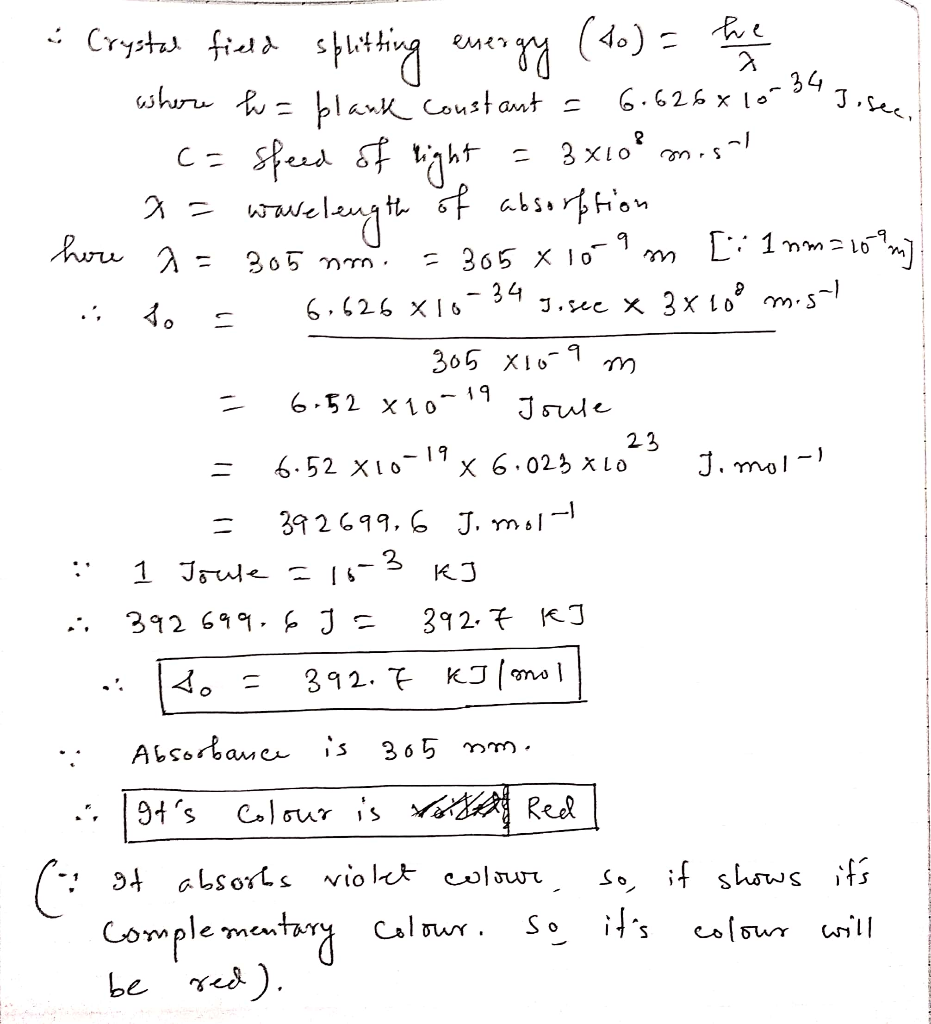#### Earn Coins

Coins can be redeemed for fabulous gifts.

Similar Homework Help Questions
• ### The complex [Fe(H2O)6]3+ has a maximum absorbance at 724 nm. a.Calculate the crystal field splitting energy,...

The complex [Fe(H2O)6]3+ has a maximum absorbance at 724 nm. a.Calculate the crystal field splitting energy, in kJ, for the complex. b.Predict the colour of the complex.

• ### CE IZOLIUM The (RhC16]has its maximum absorbance at 495 nm. 1. Calculate the crystal field splitting...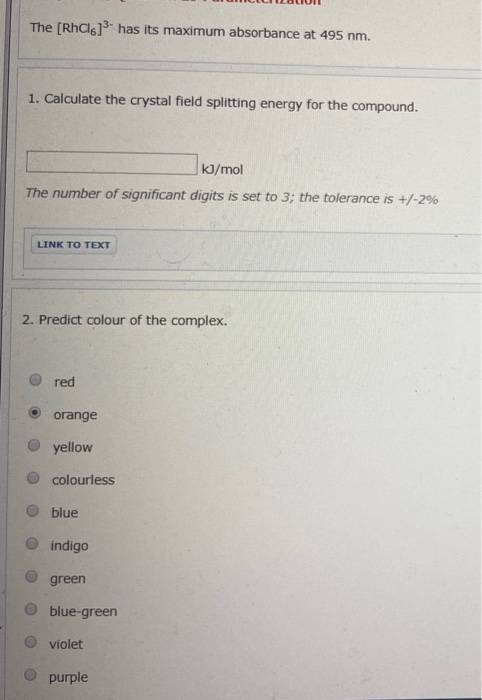CE IZOLIUM The (RhC16]has its maximum absorbance at 495 nm. 1. Calculate the crystal field splitting energy for the compound. kJ/mol The number of significant digits is set to 3; the tolerance is +/-2% LINK TO TEXT 2. Predict colour of the complex. red orange yellow O colourless O blue indigo O green blue-green violet O purple

• ### The [CrCl_6]^3- ion has a maximum in its absorption spectrum at 735 nm. Calculate the crystal...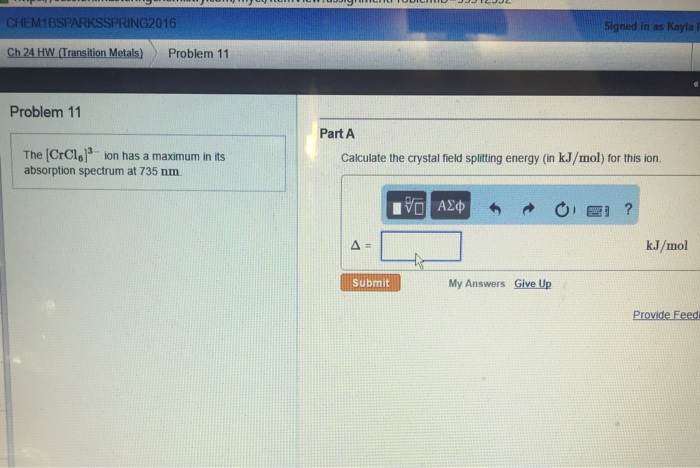The [CrCl_6]^3- ion has a maximum in its absorption spectrum at 735 nm. Calculate the crystal field splitting energy (in kJ/mol) for this ion.

• ### D orbital splitting diagram? Fe(CN)6 4-

What is the octahedral crystal-field splitting diagram for the metal Fe(CN)6 4-?Thank you.

• ### A ?1 octahedral complex is found to absorb visible light, with the absorption maximum occurring at 499 nm . Calculate the crystal-field splitting energy, Δ , in kJ/mol.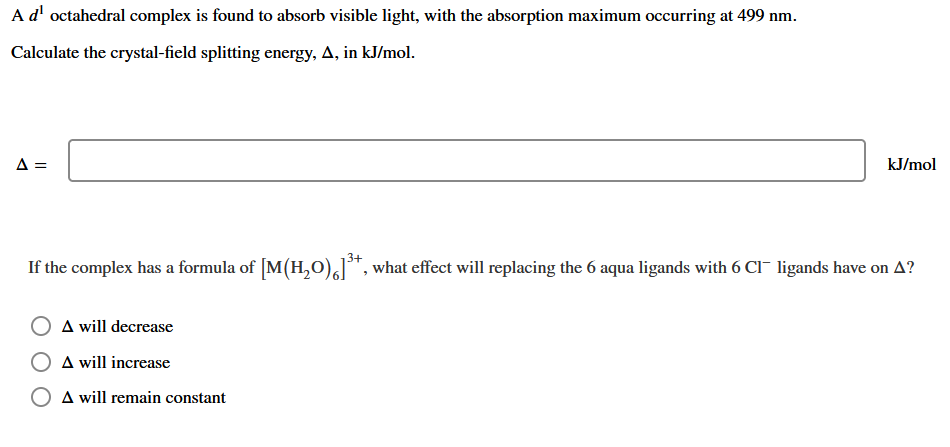A ?1 octahedral complex is found to absorb visible light, with the absorption maximum occurring at 499 nmCalculate the crystal-field splitting energy, Δin kJ/mol.

• ### Which of the following complexes has the largest crystal field splitting of the d orbitals? A....

Which of the following complexes has the largest crystal field splitting of the d orbitals? A. [Fe(H2O)6]3+ B. [FeF6]3– C. [Fe(NH3)6]3+ D. [FeI6]3– E. [Fe(CO)6]3+

• ### .. Fe is identified by the rapid formation of the intensely colored pentaaquathio-cyanatoiron (III) complex ion,...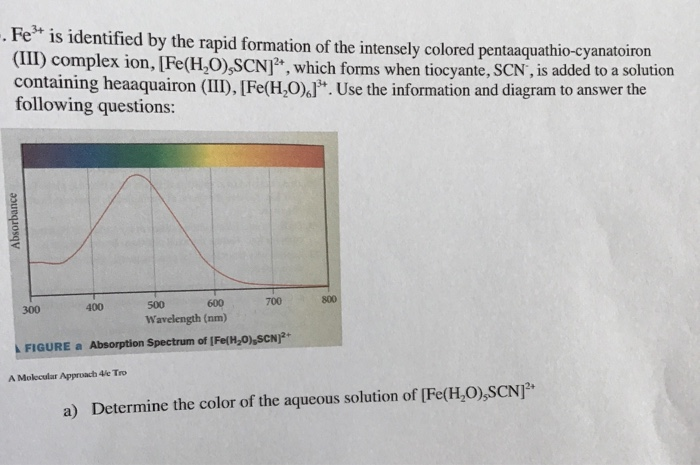.. Fe is identified by the rapid formation of the intensely colored pentaaquathio-cyanatoiron (III) complex ion, [Fe(H,O),SCN , which forms when tiocyante, SCN, is added to a solution containing heaaquairon (III), [Fe(H,0).J. Use the information and diagram to answer the following questions: Absorbance 300 400 500 600 700 Wavelength (nm) FIGURE a Absorption Spectrum of [Fe(H20),SCN)2+ A Molecular Approach 4le Tro a) Determine the color of the aqueous solution of [Fe(HO),SCN b) Calculate the crystal field splitting energy of [Fe(H,0),SCN]...

• ### The absorbance of an iron thiocyanate solution containing 0.005 mg Fe/mL was reported as 0.43 at...

The absorbance of an iron thiocyanate solution containing 0.005 mg Fe/mL was reported as 0.43 at 540 nm using a 1.00 cm cuvette. What will be the absorbance if the solution is diluted to twice its original volume and is placed in a 2 cm cuvette? Write answer to three decimal places.

• ### Consider Fe^2+, Fe^3+, and Zn^2+: a. Write the electron configuration for each ion. b. Explain why...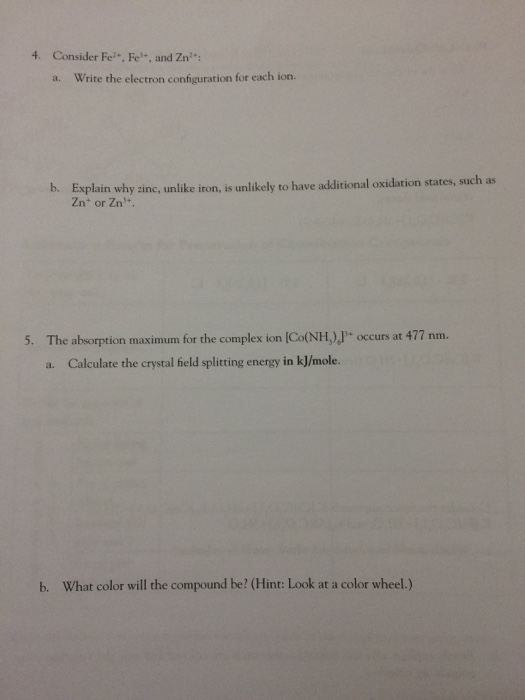Consider Fe^2+, Fe^3+, and Zn^2+: a. Write the electron configuration for each ion. b. Explain why zinc, unlike iron, is unlikely to have additional oxidation states, such as Zn^+ or Zn^3+. 5. The absorption maximum for the complex ion [Co(NH_5)_6]^3+ occurs at 477 nm. a. Calculate the crystal held splitting energy in kj/mole. b. What color will the compound be?

• ### 3. When Cl2(g) is added to aqueous K [Fe(CN)6], [Fe(CN).]*- is oxidized to [Fe(CN).]}-and K [Fe(CN)6]...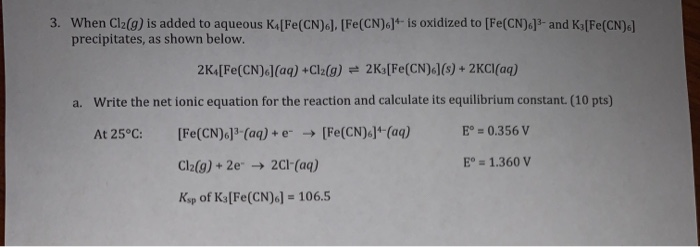3. When Cl2(g) is added to aqueous K [Fe(CN)6], [Fe(CN).]*- is oxidized to [Fe(CN).]}-and K [Fe(CN)6] precipitates, as shown below. 2K [Fe(CN).] (aq) + Cl2(g) = 2K3[Fe(CN).J(8) + 2Cl(aq) a. Write the net ionic equation for the reaction and calculate its equilibrium constant (10 pts) At 25°C: [Fe(CN).]" (aq) + e[Fe(CN).]" (aq) E° = 0.356 V Cl2(9) + 2e → 2Cl(aq) E° = 1.360 V Ksp of K3 [Fe(CN)6] = 106.5 b. When 80.0 mL of 1.00 M KCN is...